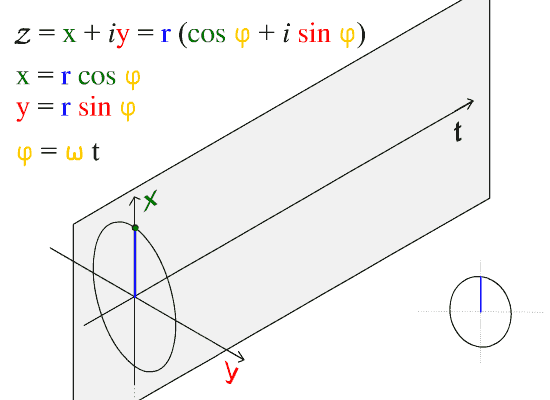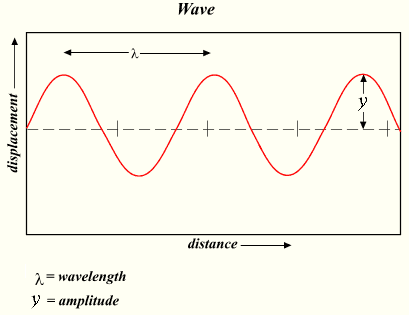Categories

# Transverse Waves

Transverse waves propagate through media with a speed →vw orthogonally to the direction of energy transfer.

A transverse wave is a moving wave that consists of oscillations occurring perpendicular (or right angled) to the direction of energy transfer. If a transverse wave is moving in the positive x-direction, its oscillations are in up and down directions that lie in the y–z plane. Light is an example of a transverse wave. For transverse waves in matter, the displacement of the medium is perpendicular to the direction of propagation of the wave. A ripple on a pond and a wave on a string are easily visualized transverse waves.

Transverse waves are waves that are oscillating perpendicularly to the direction of propagation. If you anchor one end of a ribbon or string and hold the other end in your hand, you can create transverse waves by moving your hand up and down. Notice though, that you can also launch waves by moving your hand side-to-side. This is an important point. There are two independent directions in which wave motion can occur. In this case, these are the y and z directions mentioned above. depicts the motion of a transverse wave. Here we observe that the wave is moving in and oscillating in the x-y plane.

A wave can be thought as comprising many particles (as seen in the figure) which oscillate up and down. In the figure we observe this motion to be in x-y plane (denoted by the red line in the figure). As time passes the oscillations are separated by units of time. The result of this separation is the sine curve we expect when we plot position versus time.Sine Wave: The direction of propagation of this wave is along the t axis.

When a wave travels through a medium–i.e., air, water, etc., or the standard reference medium (vacuum)–it does so at a given speed: this is called the speed of propagation. The speed at which the wave propagates is denoted and can be found using the following formula:

v=fλv=fλ

where v is the speed of the wave, f is the frequency , and is the wavelength. The wavelength spans crest to crest while the amplitude is 1/2 the total distance from crest to trough. Transverse waves have their applications in many areas of physics.

Examples of transverse waves include seismic S (secondary) waves, and the motion of the electric (E) and magnetic (M) fields in an electromagnetic plane waves, which both oscillate perpendicularly to each other as well as to the direction of energy transfer. Therefore an electromagnetic wave consists of two transverse waves, visible light being an example of an electromagnetic wave.Wavelength and Amplitude: The wavelength is the distance between adjacent crests. The amplitude is the 1/2 the distance from crest to trough.

Two Types of Waves: Longitudinal vs. Transverse: Even ocean waves!